Next: Photo-Ionization Up: Time-Dependent Perturbation Theory Previous: Magnetic Dipole Transitions

# Electric Quadrupole Transitions

According to Equation (8.195), the quantity that mediates spontaneous electric quadrupole transitions is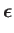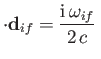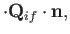(8.212)

where(8.213)

is the electric quadrupole matrix element. It follows, by analogy with with Equation (8.165), that the spontaneous emission rate associated with an electric quarrupole transition is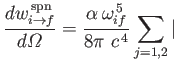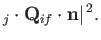(8.214)

Here,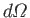is the solid angle associated with the direction of the emitted photon's normalized wavevector,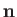. Moreover,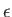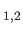are the photon's two independent electric polarization vectors.

The selection rules for electric quadrupole transitions in a hydrogen-like atom are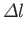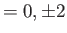(8.215)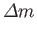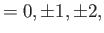(8.216)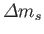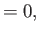(8.217)

whereis the azimuthal quantum number,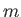the magnetic quantum number, and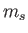the spin quantum number. A more general form of these selection rules is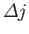(8.218)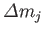(8.219)

where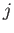and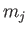are the standard quantum numbers associated with the total angular momentum of the system. Note, however, that electric quadrupole transitions between two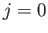states, or between two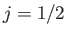states, or between a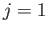state and astate, are forbidden .

Let us estimate the typical spontaneous emission rate for an electric quadrupole transition in a hydrogen atom. We expect the matrix element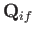, defined in Equation (8.216), to be of order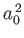, where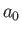is the Bohr radius. We also expect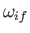to be of order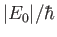, where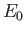is the hydrogen ground-state energy. It thus follows from Equation (8.217) that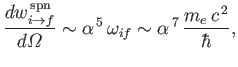(8.220)

which is of order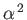times smaller than a typical electric dipole transition rate. [See Equations (8.180).]Next: Photo-Ionization Up: Time-Dependent Perturbation Theory Previous: Magnetic Dipole Transitions
Richard Fitzpatrick 2016-01-22